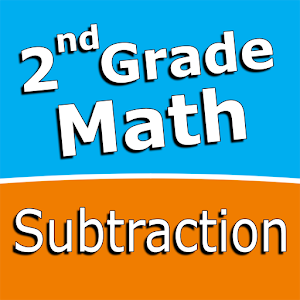Español

Todos los días ofrecemos apliaciones y juegos licenciados GRATIS para Android de otra manera tendrás que comprarlo.\$2.99
EXPIRÓ

# Android Giveaway of the Day - Second grade Math - Subtraction

Intuitive interface powered by handwriting input and a fun and engaging mini game.
\$2.99 EXPIRÓ
Votación de Usuario: 1

Esta oferta de regalo ha expirado. Second grade Math - Subtraction ya está disponible.

Intuitive interface powered by handwriting input and a fun and engaging mini game in addition to a regular math trainer mode make our app stand out from the crowd of generic math learning apps.

With Second grade Math - Subtraction you can practice and improve the following math skills:
- Subtraction facts - numbers up to 20
- Subtract a one-digit number from a two-digit number
- Subtract two two-digit numbers
- Subtract multiples of 10
- Subtract multiples of 100
- Subtract multiples of 10 or 100
- Subtract three-digit numbers
- Balance subtraction equations

Sergey Malugin

Education

### Versión:

Varies with device

### Tamaño:

Varies with device

Everyone